# XGBoost with cWB¶

Library - XGBoost integrated in cWB: GIT repository
XGBoost - version 1.4.2: GIT repository
Quick Source - Preferably should set up your own cWB branch and virtual environment with XGBoost … for the example discussed below source the following:
source /home/tanmaya.mishra/GIT/GV_XGB_DEV/library/cit_watenv_tm.sh
source /home/waveburst/virtualenv/xgboost/bin/activate


References

What is eXtreme Gradient Boosting (XGBoost)

 Introduction: eXtreme Gradient Boosting Why XGBoost?: Veto Method Limitations

Enhancing cWB with XGBoost

 Method: Overall idea for integration of XGBoost into cWB cWB Summary Statistics: List of input features (cWB summary statistics) for XGBoost Reduced Detection Statistic: XGBoost Penalty Factor

XGBoost Model Implementation ->

 Data Splitting: Setting up cWB working directories, data splitting for XGBoost training and testing Tuning: Finding the most optimal XGBoost hyper-parameters set Training: Creating and Storing the XGBoost model Testing: Predicting and estimating the significance using the stored XGBoost model Report: Creating the final report page and additional plotting options

## XGBoost - eXtreme Gradient Boosting¶

### Introduction¶

Our goal is to improve the sensitivity of the cWB search to binary black hole (BBH) mergers by using Machine Learning (ML) algoithms. Traditional ML algorithms e.g. support vector machines, nearest neighbor algorithms, and decision tree based algorithms are typically applicable when the data is pre-processed and the dimensionality of the data is limited. Deep learning methods, on the other hand, are generally suitable for processing raw, high-dimensional data.
XGBoost aka eXtreme Gradient Boosting is an ensemble learning, decision-tree based, supervised ML algorithm. In XGBoost, instead of using a single decision tree to classify events, an ensemble of decision trees is generated. A decision tree is used as the base learner, and subsequent learners (trees) are formed based on the residual errors obtained after each iteration (boosting). The misclassified instances from each tree (residual) is weighted and input into the subsequent tree as shown in the Figure below.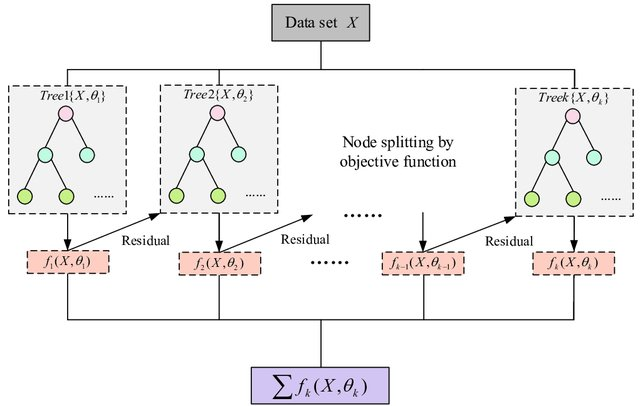• XGBoost flow chart for building an ensemble of trees. For a given dataset X, XGBoost builds subsequent trees by learning the residual of the previous tree as the input for the next tree [image credit].

The output of a decision tree is given by the leaf score (estimated by the Log-Odds output) which helps in determining the category of a given observation. In XGBoost Classifier, the leaf scores from all the decision trees in the ensemble are added together to get a margin score. The final output $$P_{XGB}$$ is computed by taking the sigmoid of the margin score, where a value close to zero denotes a noise-like event and a value close to one denotes a signal-like event.

### Why XGBoost?¶

GW detector data contains non-Gaussian noise artifacts known as glitches, some of the noise events are reconstructed by the cWB pipeline and pollute the data used for analysis. In the standard cWB analysis, a series of a priori defined veto thresholds are applied on a list of cWB summary statistics to target and remove these glitches. This approach, henceforth known as the veto method, improves the significance of candidate GW events by removing noise events and reducing excess background.

Veto method and its limitations

The veto method classifies reconstructed events into one of the two categories: signal-like events and noise-like events. Events that fall into the noise-like category are removed from the analysis. While this method works well, it inevitably results in discarding borderline GW events which do not pass the veto thresholds and at the same time makes the pipeline vulnerable to the high SNR glitches which do pass the vetoes. Designing vetoes manually in the multidimensional space of the summary statistics is challenging, and furthermore, requires re-tuning of the veto thresholds for each detector network configuration and each observing run.
Binary classification is a standard problem in the ML literature with many prominent ML algorithms based on the decision-tree structure.

XGBoost Classifier

We find that XGBoost is the most suitable choice of ML algorithm for the cWB classification problem. Morever, XGBoost is computationally efficient and the model training and testing procedures are completed within minutes using one CPU core.

## Enhancing cWB with XGBoost¶

### Method¶

XGBoost improves the sensitivity of the cWB search to binary black hole mergers while replacing the veto method. In order to construct the XGBoost model, we carefully select a subset of 14 summary statistics estimated by cWB as the input features for the ML algorithm. This is done by looking at the correlation among the summary statistics by using the Spearman Correlation Coefficient and picking the summary statistics that have the least correlation with each other. We use the accumulated background data and generate signal events (simulations) data to train and test our supervised XGBoost algorithm.
The XGBoost hyper-parameter values are optimized specifically to prevent overfitting by tuning over six standard XGBoost hyper-parameters using a grid search with 10-fold cross-validation, with respect to the precision-recall area under the curve (AUC PR) metric. A separate ML model is trained for each search configuration and each observing run. For training, we select 100 yr of background data per data chunk. For every 100 yr of background data we select approximately 1000 simulation events. Once trained, the ML model predictions are directly incorporated into the standard cWB search’s detection statistic $$\eta_0$$ to obtain the reduced detection statistic $$\eta_r$$.
The list of cWB summary statistics that are used as the input features for the XGBoost model are described in cWB Summary Statistics. Subsequent testing showed that adding supplementary statistics contributed redundant information and did not markedly improve classification. On the other hand, further feature pruning led to diminished classification.

### Reduced Detection Statistic¶

The standard cWB detection $$eta_{{0}}$$ is updated to be more sensitive to the $$chi^2$$ factor as follows:

$\eta_{0} = \sqrt{\frac{E_{c}}{1+\chi^2(\text{max}(1, \chi^2)-1)}}$

We incorporate the predictions made by the ML model directly into the detection statistic to improve noise rejection. We define the reduced detection statistic used for the ML-enhanced cWB search as:

$\eta_{r} = \eta_{0}\cdot W_{{XGB}},$

where $$W_{{XGB}}$$ is the XGBoost penalty factor. To compute $$W_{{XGB}}$$, we first apply a correction to the XGBoost output $$P_{{XGB}}$$, defined in Mishra et al. 2021. This correction is designed to suppress numerous noise events having less than one cycle in the time domain waveform, typical for the known family of glitches found in the GW detector data. Next, we apply a monotonic transformation, defined in Mishra et al. 2021, to obtain the penalty factor $$W_{{XGB}} = W_{{XGB}}(P_{{XGB}})$$. This transformation accentuates the ranking of events with the values of $$P_{{XGB}}$$ very close to unity.
Although $$W_{{XGB}}$$ itself could be used as a detection statistic, we find that it is susceptible to assigning high significance to low SNR noise events. Instead, we use it as a penalty factor to the estimated effective correlated SNR $$\eta_{0}$$. The end result is a detection statistic $$\eta_{r}$$ which is enhanced by the ML classification and resistant to overfitting the low SNR noise events.

## XGBoost model implementation ->¶

The XGBoost framework has been successfully integrated with the cWB pipeline and different XGBoost utilities can be accessed with cwb commands. A new cWB command has been added cwb_xgboost (details can be found in the commands page).
The following sections show how to implement the XGBoost method for the BBH/IMBH search configurations. Separate sections on different searches will be added soon ..

### Data Splitting¶

In order to train and test the XGBoost model, we start by splitting the available data (both background and simulation) into two parts: Training data and Testing data.

Setting up the working directories

• We start by setting up 3 directories:
• BKG - To store the Background data for each chunk involved in the XGBoost model.
• SIM - To store the Simulations data.
• XGB - To store the trained XGBoost model.
• Clone working directories for XGBoost use by utilizing the cwb_clonedir command (for simulation directories add option ‘ –simulation true’ while cloning). This step is optional; XGBoost analysis can be performed in the original working directories as well.
cwb_clonedir /in_path_BKG/BKG_dir   /out_path/BKG/BKG_dir_xgb1   '--output smerge --label M1.V_hvetoLH'
cwb_clonedir /in_path_SIM/SIM_dir   /out_path/SIM/SIM_dir_xgb1   '--output smerge --label M1.C_U.V_hvetoLH --simulation true'

• Create the XGB directory using cwb_mkdir /out_path/XGB/Model_dir_XGB.

XGB cuts

• Frequency cuts are applied prior to XGBoost processing. The frequeny cuts are different for different search configurations:
• BBH configuration: 60 Hz < $$f_0$$ < 300 Hz
• IMBH configuration: $$f_0$$ < 200 Hz
• BurstLF configuration: 24 Hz < $$f_0$$ < 996 Hz
• The wave root files get a label xgb_cut at the end to denote that the frequency cut has been applied on those files.
cwb_setcuts M1.V_hvetoLH '--wcuts ((frequency>60&&frequency<300)&&(!veto_hveto_H1&&!veto_hveto_L1)) --label xgb_cut'


Data split

• We split the data for training and testing.
• BKG data - For each chunk of available background data (usually around a 1000 years unless the chunk hosts some special GW events), 100 years of background data is kept for training, and the remaining data is set aside for testing.
• SIM data - For every 100 years of background data that is used for training, we split 1000 simulation events for training and the keep the rest of the simulation events for testing purpses.
• The datasplit option is utilized for splitting the data with an option –ifrac which allows us to choose whether we want to split the root files based on number of years for BKG/events for SIM (–ifrac -x, where x is an integer and x > 1) or based on the fraction of root events stored (–ifrac y, where 0<y<1).
cwb_xgboost datasplit '--mlabel M1.V_hvetoLH.C_xgb_cut --ifrac -100 --verbose true --cfile $CWB_CONFIG/O3a/CHUNKS/BBH//Chunk_List.lst'  • The –cfile option points to the GPS times for the chunks available in order to assign the respective chunk numbers $$C_n$$. • After the split we end up with two new wave root files for training and testing purposes. • Create the following files in the XGB directory for tuning and training: • nfile.py - Input background files (wave_*.root/.py) for training. • sfile.py - Input simulation files (wave_*.root/.py) for training. Note cwb_xgboost_config.py script is used as the default input XGBoost configuration for tuning/training/prediction/report functionalities of the cwb_xgboost command. The default config values and options can be changed by including user defined user_xgboost_config_ .py files in the cwb_xgboost command options. The cwb_xgboost_config.py script is included at the end of this page for completion. ### Tuning¶ XGBoost has a number of free hyper-parameters which control various properties of the learning process to prevent overfitting. These hyper-parameters need to be tuned for each specific application. Some primary XGBoost hyper-parameters are fixed e.g. objective: binary:logistic - This is the cost function used by the XGBoost Classifier, tree_method: hist - Tells the algorithm to build trees using distributed training, grow_policy: lossguide - the split is made at the node with the highest loss change, n_estimators: 20,000* parameter determines the total number of generated trees in the model. * A method known as early stopping is employed to optimize the total number of trees generated. In early stopping, a small fraction of the training data set is set aside for validation. When the validation AUC PR score stops improving, the training ends to prevent the XGBoost from overfitting. We perform a grid search over a range of six standard XGBoost hyper-parameters, listed below. We find the most optimal set by evaluating each configuration of XGBoost hyper-parameters with respect to the precision-recall area under the curve (AUC PR) over 10-fold cross-validation. The optimal configuration of hyper-parameters, according to this criteria, is shown in bold.  XGBoost hyper-parameters entry learning_rate 0.03, 0.1 max_depth 13 min_child_weight 10.0 colsample_bytree 1.0 subsample 0.4, 0.6, 0.8 gamma 2.0, 5.0, 10.0 Here, the learning_rate parameter regulates how much each generated tree effects the final prediction. The max_depth parameter determines the deepness of each tree and thus effects the overall complexity of the model. Other parameters (min_child_weight, colsample_bytree, subsample, gamma) act as different forms of regularization to preserve the conservativeness of the algorithm and prevent overfitting. We use around 20% of the training data for tuning. Sample Weight options • Apart from the XGBoost hyper-parameters, there are some user defined hyper-parameters that control the shape of a custom $$\eta_0$$ dependent sample weight which is applied on the background events to minimize the importance given by the algorithm to low SNR glitches. All the simulation events are assigned a sample weight of 1. In contrast, for the noise events, we first divide simulation events in the interval 6.5<$$\eta_{0}$$<20 into $$nbins$$ = 100 percentile bins such that the number of simulation events in each bin is the same. The lower threshold at $$\eta_0$$ = 6.5 gets rid of excess background with minimal or no loss of simulated events. The capping at $$\eta_0$$ = 20.0 prevents the algorithm from being affected by high SNR background events. • Sample weight $$w$$: For simulation events - $$w_{S}$$ = 1. For background events - $w_{B}(i) = \frac{N_{S}(i)}{N_{B}(i)}, e^{ln(A)(1-\frac{i}{nbins})^q}$ where, $$i = {1,2, …, nbins}$$ is a given bin, $$N_{S}(i)$$ and $$N_{B}(i)$$ are the number of simulation and background events in the $$i^{{th}}$$ bin. ($$q, A$$) are weight options where $$A$$ is called the balance parameter $$A=\frac{N_{S}(1)}{N_{B}(1)}$$ is the class balance ($$N_{S}/N_{B}$$) for the first bin at $$\eta_{0} \geq 6.5$$, $$q$$ is called the slope parameter that controls the rate of change of weighted background distribution (defined in Mishra et al. 2022). For all events with $$\eta_{0} \geq 20$$, the number of simulation events is re-sampled to match the number of background events to have a perfect class balance ($$N_{S}/N_{B} = 1$$). For a given combination of ($$q, A$$) values, we can achieve any monotonic distribution of our choice.  XGBoost hyper-parameters entry weight balance $$A$$ = 40 weight slope $$q$$ = 5 • The user_pparameters.C file is modified for tuning and the commands for tuning are as follows: cwb_condor xgb r1 cwb_xgboost report '--type tuning --ulabel r1 --verbose true'  Show/Hide user_pparameters.C file user_pparameters.C - // ---------------------------------------------------------- // XGB parameters used for Tuning // ---------------------------------------------------------- // This is an example which shows how to setup XGB in the user_pparameters.C file { // tuning/training/prediction pp_xgb_search = "bbh"; // input search type (bbh/imbhb/blf/bhf/bld) // tuning pp_xgb_ofile = "xgb/r1/tuning.out"; // output XGB hyper-parameters file // tuning/training pp_xgb_nfile = TString(work_dir)+"/nfile.py"; // input background file (wave_*.root/.py) pp_xgb_sfile = TString(work_dir)+"/sfile.py"; // input simulation file (wave_*.root/.py) pp_xgb_nfrac = 0.2; // input fraction of events selected from bkg pp_xgb_sfrac = 0.2; // input fraction of events selected from sim // pp_xgb_config = "$CWB_SCRIPTS/cwb_xgboost_config.py"; // input XGB configuration
// if not provided then the default config cwb_xgboost_config.py is used

pp_xgb_config         = "config/user_xgboost_config_r1.py";   // input XGB configuration, leave empty if there are no changes needed

// XGB hyper-parameters (tuning)

// r1
pp_xgb_learning_rate    = { 0.03 };
pp_xgb_max_depth        = { 13 };
pp_xgb_min_child_weight = { 10.0 };
pp_xgb_colsample_bytree = { 1.0 };
pp_xgb_subsample        = { 0.6 };
pp_xgb_gamma            = { 2.0 };
pp_xgb_balance_balance = { "A=1", "A=5", "A=20", "A=40", "A=100", "A=300", "A=500" };
pp_xgb_balance_slope = { "q=0.5", "q=0.75", "q=1.0", "q=1.5", "q=2.0", "q=2.5", "q=3.0", "q=5.0", "q=10.0" };
pp_xgb_caps_rho0 = { 20 };

// used as input by the "cwb_condor xgb" command
// can be also defined in user_parameters.C file
strcpy(condor_tag,"ligo.prod.o3.burst.bbh.cwboffline");

}


### Training¶

Training is done in the /out_path/XGB/model_dir_XGB directory. Create the following config file user_xgboost_config_v1.py in the config folder in the XGB model directory:
/out_path/XGB/model_dir_XGB/config/user_xgboost_config_v1.py


Show/Hide user_xgboost_config_v1.py file

 ML_caps['rho0'] =  20                          #set the cap value for rho0 or eta_0, can be used to set cap value for other summary statistics
ML_balance['slope(training)']     = 'q=5'      #set the weight slope hyper-parameter
ML_balance['balance(training)']   = 'A=40'     #set the weight balance hyper-parameter

ML_list.remove('Lveto2')                       #remove any cWB summary statistic from the input features list

import cwb_xgboost as cwb
ML_list.append(cwb.getcapname('norm',13))      #add any cWB summary statistic along with cap value for that statistic

ML_options['rho0(mplot*d)']['enable'] = True   #1d and 2d hist plots
ML_options['Qa(mplot*d)']['enable'] = True
ML_options['Qp(mplot*d)']['enable'] = True     #similarly for other summary statistics

ML_options['bkg(mplot2d)']['rho_thr'] = 15     #bkg minimum rho0 threshold for plotting on 2d hist

ML_options['sim(mplot2d)']['cmap'] = 'rainbow' #color map for sim in the 2d hist


Once the config file is setup as per the requirements, the XGBoost model is trained by running the following command in the XGB model directory:
cwb_xgboost training '--nfile nfile.py --sfile sfile.py  --model xgb/v1/xgb_model_name_v1.dat --nfrac 1.0 --sfrac 1.0 --search bbh --verbose true --dump true --config config/user_xgboost_config_v1.py'
cwb_mkhtml xgb/v1/                     //(plots stored in public_html training report page in ldas)

After training is completed, the trained model is stored as /out_path/XGB/model_dir_XGB/xgb/v1/xgb_model_name_v1.dat and the training information and plots can be found in the training report page.

A few example plots stored in the training report page

Show/Hide training report page plots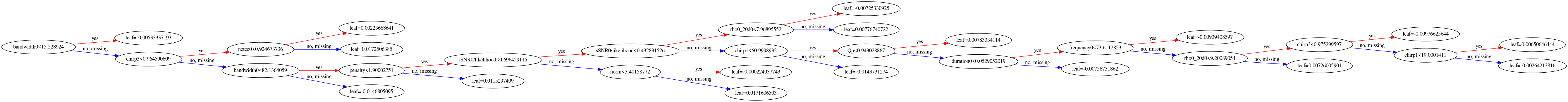• Last Tree Generated - Illustration of the last tree generated in the ensemble before training procedure stops.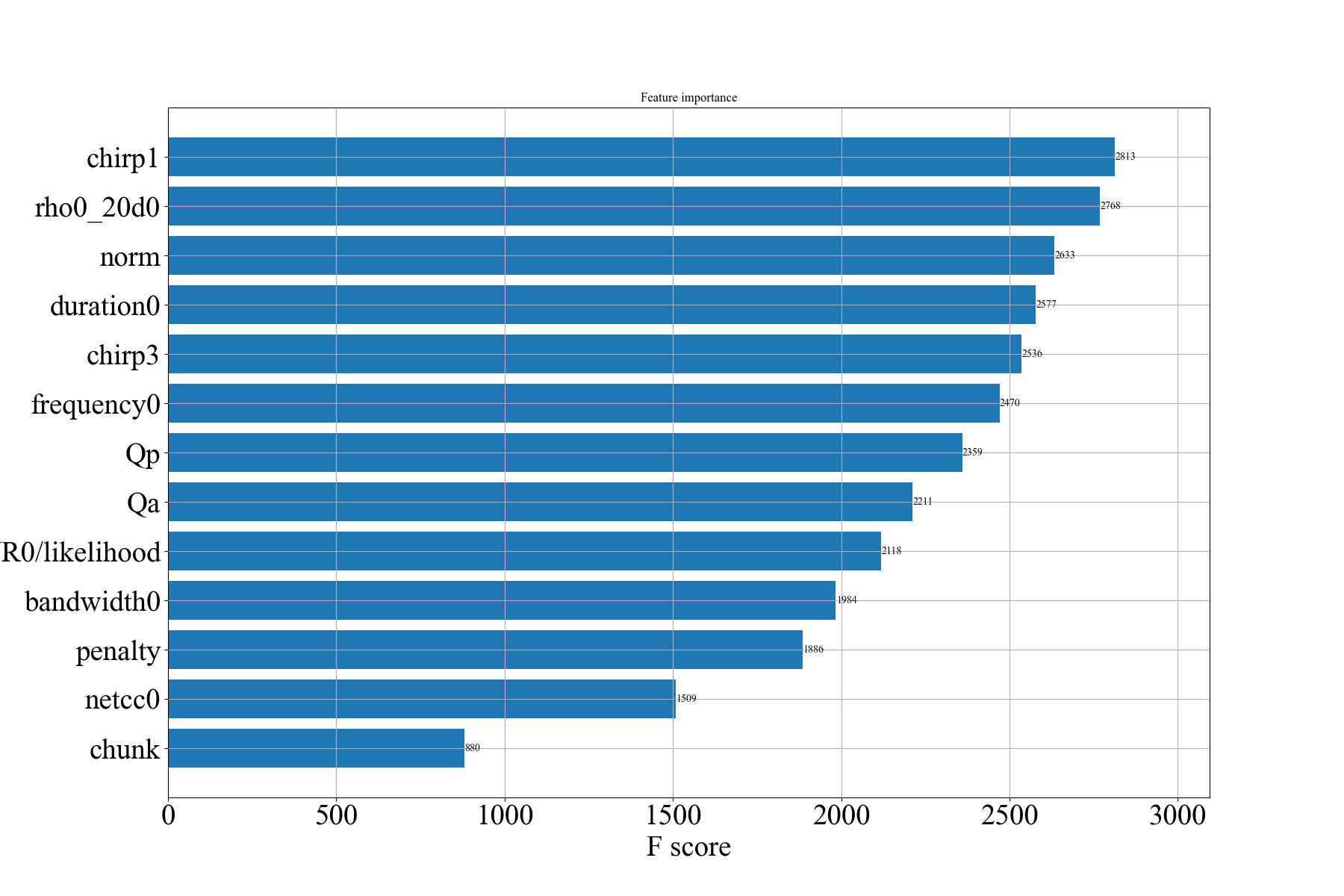• Feature importances - Importance of input features/summary statistics in training the XGBoost model.• $$Q_a$$ vs $$Q_p$$ training plot - 2d hist plot of $$Q_a Q_p$$ with scatterplot of high SNR background events.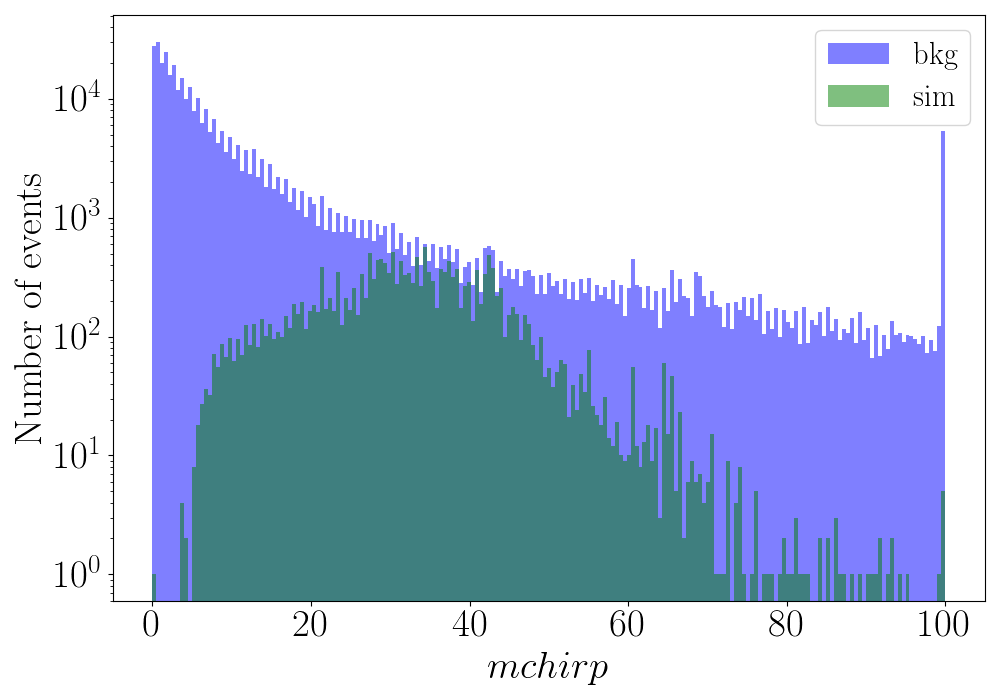• Chirp mass $$\mathcal{M}$$ - Distribution of $$\mathcal{M}$$ or mchirp for training background and simulation events.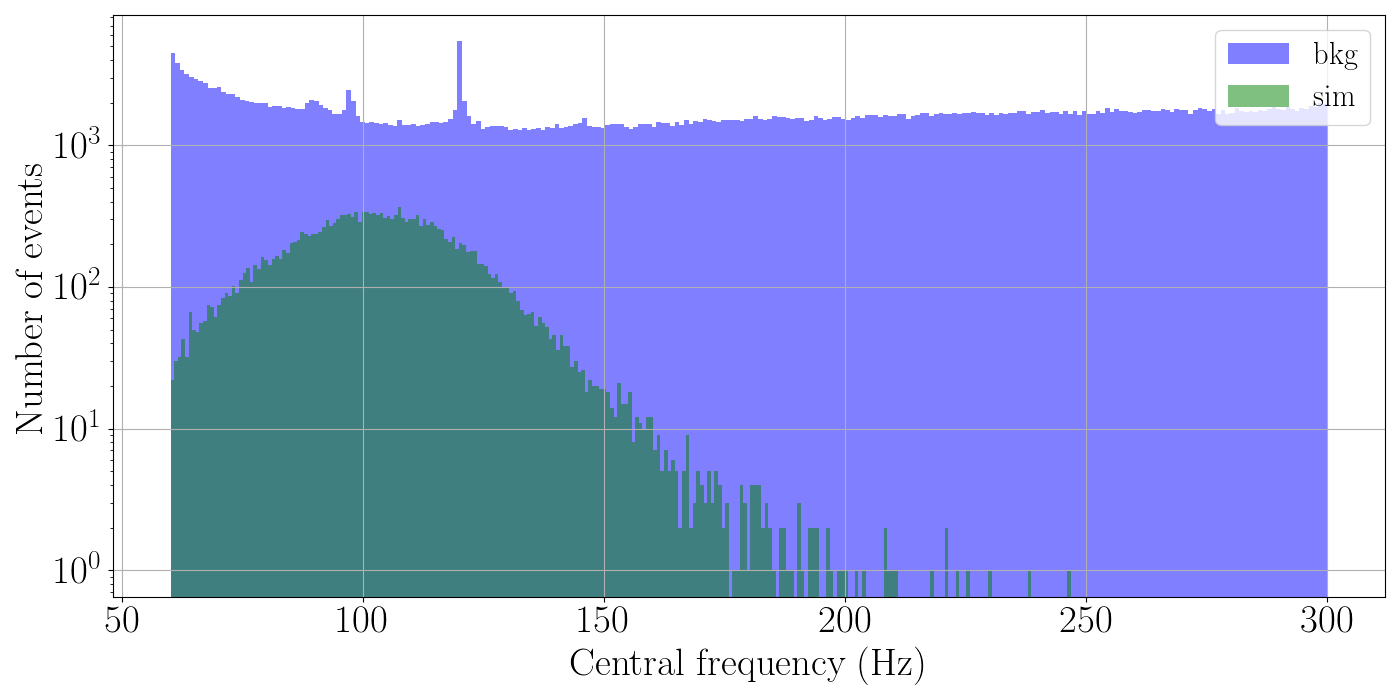• Central frequency $$f_0$$ - Distribution of central frequency $$f_0$$ for training background and simulation events.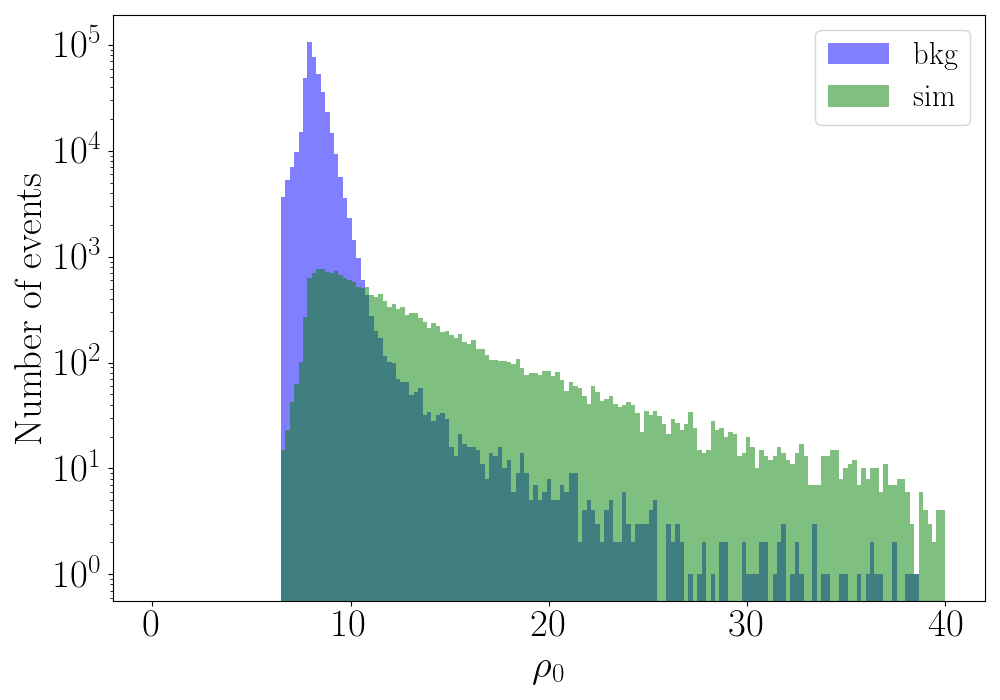• $$\eta_0$$ - Distribution of $$\eta_0$$ or $$\rho_0$$ for training background and simulation events.

### Testing¶

The trained XGBoost model is now called to predict the $$W_{XGB}$$ for a given cWB event and subsequently estimating the reduced detection statistic $$\eta_r$$. The $$\eta_r$$ statistic is then used to assign significance to simulation events based on the background rate distribution, and conduct further analysis. The testing/preiction procedure is as follows:

BACKGROUND

• /out_path/BKG/BKG_dir_xgb1 - For each chunk of background data, we go to the respective working directory and run the following commands:

Standard Veto Method for comparison

Set the standard PP cuts/veto method for the testing wave root file and create a standard cWB report.
cwb_setcuts M1.V_hvetoLH.C_xgb_cut.XK_Test_Y100_TXXXX '--tcuts bin1_cut --label bin1_cut'
cwb_report M1.V_hvetoLH.C_xgb_cut.XK_Test_Y100_TXXXX.C_bin1_cut create

Collect the background root files after standard PP cuts application in nfile_bkg_std.py file for testing with XGBoost.

Prediction with XGBoost

Calling the stored XGBoost model for prediction and then creating standard cWB report page.
cwb_xgboost M1.V_hvetoLH.C_xgb_cut.XK_Test_Y100_TXXXX '--model /out_path/XGB/model_dir_XGB/xgb/v1/xgb_model_name_v1.dat --ulabel v1 --rthr 0 --search bbh'
cwb_report M1.V_hvetoLH.C_xgb_cut.XK_Test_Y100_TXXXX.XGB_rthr0_v1 create

Once the prediction step is completed for all the chunks, we collect the far_rho.txt files for each chunk and store it in the far_rho_v1.lst file in a new folder /out_path/BKG/XGB/far_rho_v1.lst. Similarly, we collect the preddicted root files for each chunk in the nfile_bkg_rhor_v1.py file in the folder /out_path/BKG/XGB/nfile_bkg_rhor_v1.py.

SIMULATION

• /out_path/SIM/SIM_dir_xgb1 - For each simulation directory, we run the following commands:

Standard Veto Method for comparison

Set the standard PP cuts/veto method for the testing wave root file and set ifar.
#set standard PP cuts
cwb_setcuts M1.C_U.V_hvetoLH.C_xgb_cut.XK_Test_NXX_TXXX '--tcuts bin1_cut --label bin1_cut'

#set ifar chunkwise, far_bin1_cut_file pointing to the standard far_rho.txt files for the respective BKG chunk
cwb_setifar M1.C_U.V_hvetoLH.C_xgb_cut.XK_Test_NXX_TXXX.C_bin1_cut        '--tsel O2_K01_cut --label ifar --file far_bin1_cut_file  --mode exclusive'
cwb_setifar M1.C_U.V_hvetoLH.C_xgb_cut.XK_Test_NXX_TXXX.C_bin1_cut.S_ifar '--tsel O2_K02_cut --label ifar --file far_bin1_cut_file  --mode exclusive'
#... so on for all the chunks.


Prediction with XGBoost

We first make the following addition to the config/user_pparameters.C file in the simulation directory:
/out_path/SIM/SIM_dir_xgb1/config/user_pparameters.C


Show/Hide user_pparameters.C for setting IFAR

  // XGB
#define nCHUNKS       X
// read far vs rho background files
TString xgb_far_bin1_cut_file;
for(int n=0;n<xgb_xfile.size();n++) xgb_far_bin1_cut_file[n+1]=xgb_xfile[n];
if(xgb_xfile.size()!=nCHUNKS) {
cout << endl;
for(int n=0;n<xgb_xfile.size();n++) cout << n+1 << " " << xgb_far_bin1_cut_file[n+1] << endl;
cout << endl << "user_pparameters.C error: config/far_rho.lst files' list size must be = "
<< nCHUNKS << endl << endl;
exit(1);
}


After editing the user_pparameters.C file we proceed to the prediction for simulation events followed by setting ifar using the predicted bkg far.
#prediction
cwb_xgboost M1.C_U.V_hvetoLH.C_xgb_cut.XK_Test_NXX_TXXX '--model /out_path/XGB/model_dir_XGB/xgb/v1/xgb_model_name_v1.dat --ulabel v1 --rthr 0 --search bbh'

#set ifar
cwb_setifar M1.C_U.V_hvetoLH.C_xgb_cut.XK_Test_NXX_TXXX.XGB_rthr0_v1        '--tsel O2_K01_cut --label ifar --file xgb_far_bin1_cut_file  --mode exclusive'
cwb_setifar M1.C_U.V_hvetoLH.C_xgb_cut.XK_Test_NXX_TXXX.XGB_rthr0_v1.S_ifar '--tsel O2_K02_cut --label ifar --file xgb_far_bin1_cut_file  --mode exclusive'
#... so on for all the chunks.

Once the above step is performed for all the simulations available for the specific search, we proceed to produce the final report pages with the result plots. Make a new folder /out_path/SIM/SIM_dir_test where we store the following files:
• sfile_rhor_v1.py - list of all the predicted root files using XGBoost for all simulation directories used (reduced detection statistic, rhor or $$\eta_r$$).
• sfile_rho1.py - list of all the standard PP cuts root files for all simulation directories used (standard detection statistic, rho1 or $$\eta_1$$).

### Report¶

In order to create the final report containing the comparison and result plots, we create a report_config_v1.py file:
/out_path/SIM/SIM_dir_test/report_config_v1.py


Show/Hide report_config_v1.py for setting IFAR

#Plot the roc - detection efficiency vs far (yr)
PLOT['roc']  = ['xgb/v1/roc.png','','']
ROC['rhor'] = ['sfile_rhor_v1.py','red']
ROC['rho1'] = ['sfile_rho1.py','blue']

#Plot detetion efficiency @IFAR 1yr vs the central frequency freq
PLOT['efreq']  = ['xgb/v1/efreq.png','','',20,204,44,1]
EFREQ['rho_r'] = ['sfile_rhor_v1.py','red']
EFREQ['rho_1'] = ['sfile_rho1.py','blue']

#Plot the 1d histogram of rhor for both sim and bkg
PLOT['hrho'] = ['xgb/v1/hrhor.png','','r$\rho_r$']
HRHO['sim'] = ['sfile_rhor_v1.py',1,'green']
HRHO['bkg'] = ['/out_path/BKG/XGB/nfile_bkg_rhor_v1.py',1,'blue']

#Plot of log10(rhor) vs 0.5*log10(likelihood) with bkg points plotted for rhor> 8
PLOT['lrho'] = ['xgb/v1/lrhor.png','','r$\rho_r$']
LRHO['sim'] = ['sfile_rhor_v1.py',1,'green']
LRHO['bkg'] = ['/out_path/BKG/XGB/nfile_bkg_rhor_v1.py',1,'blue',8]

PLOT['qaqp'] = ['xgb/v1/qaqp_rthr8.png','rho1',8,'rhor',0.15,0.8]
QAQP['sim'] = ['sfile_rhor_v1.py']
QAQP['bkg'] = ['/out_path/BKG/XGB/nfile_bkg_rhor_v1.py']


XGBoost final report generation:
cwb_xgboost report  '--type prediction --subtype roc/hrho/efreq/lrho/qaqp --config report_config_v1.py '
cwb_mkhtml xgb/v1/

All the generated plots are stored in /out_path/SIM/SIM_dir_test/xgb/v1/
cwb_xgboost report contains more options and they are described in details in the cwb_xgboost command page.

Plots in the final XGBoost report page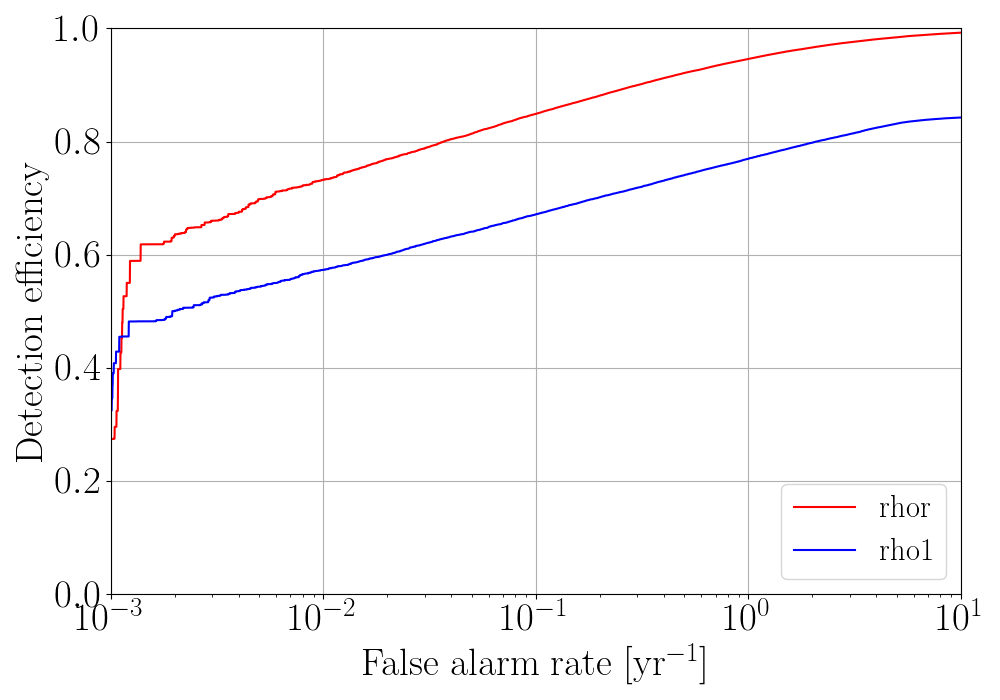• roc - Detection efficiency vs FAR plot for the ML-enhanced cWB search compared with the standard cWB search.

Show/Hide other important plots from the testing report page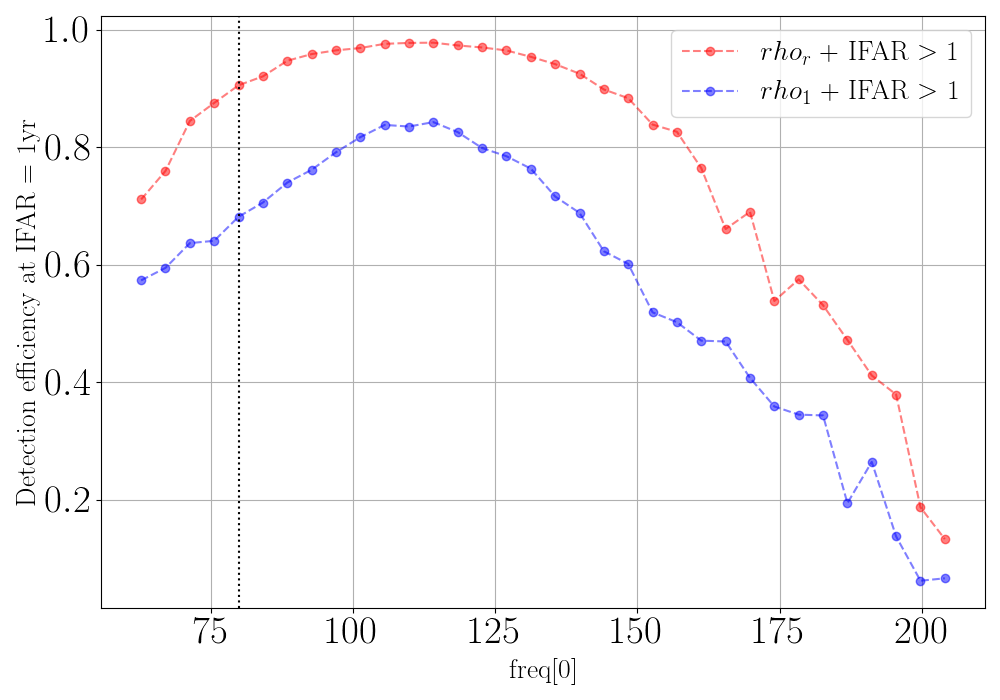• efreq - Detection efficiency @IFAR 1 yr vs central frequency $$f_0$$ (or freq) for the ML-enhanced cWB search compared with the standard cWB search.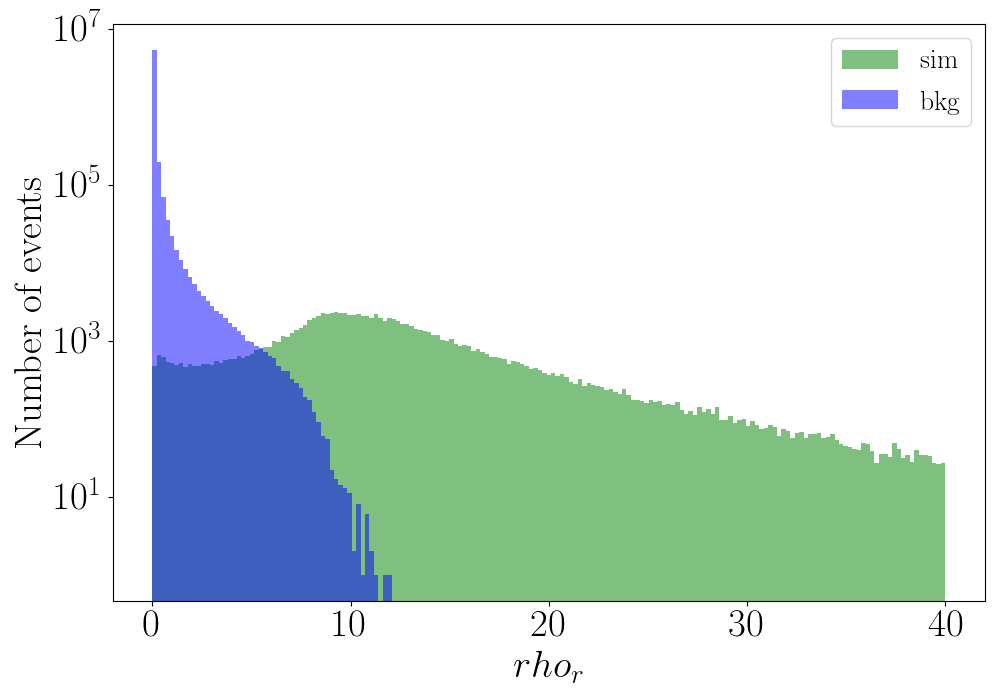• hrhor - Distribution of $$\eta_r$$ or $$rho_r$$ for testing background and simulation events.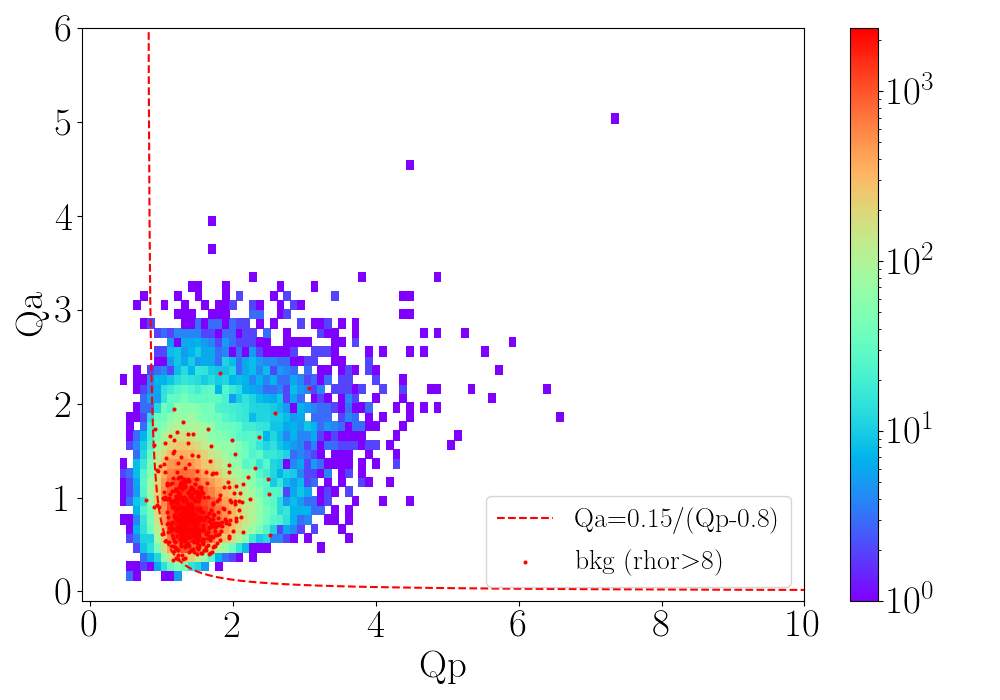• qaqp - 2d hist plot of $$Q_a$$ vs $$Q_p$$ with scatterplot of high SNR background events for testing events.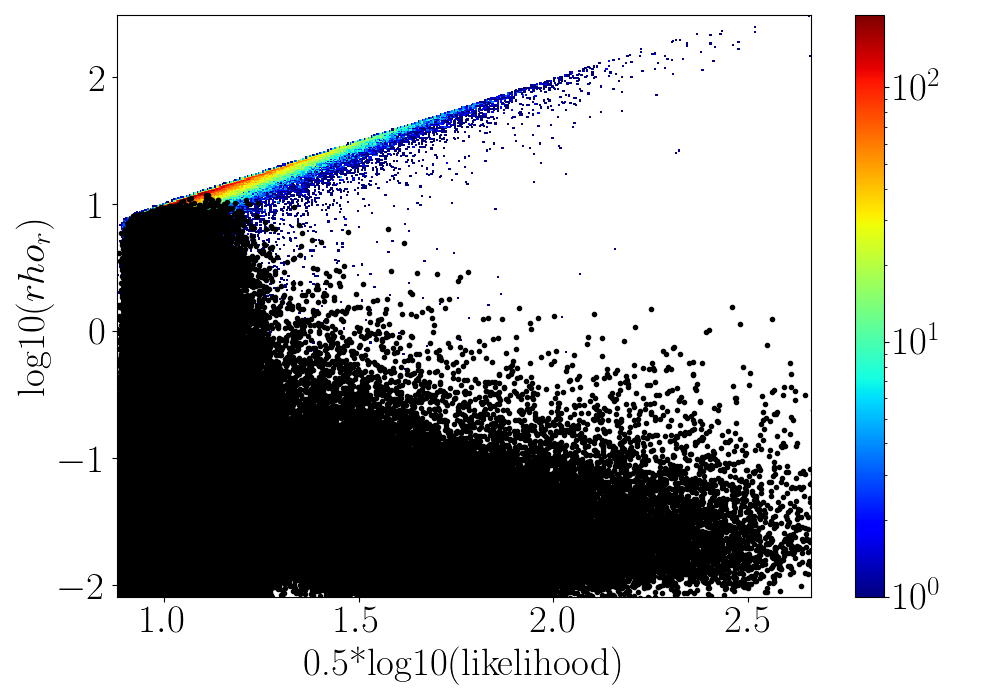• lrhor - $$log10(\eta_r)$$ or $$log10(rho_r)$$ vs $$0.5*log10(likelihood)$$ for testing simulation events and scatterplot of high SNR testing background events.

### cWB Summary Statistics¶

The following 14 cWB summary statistics are used as the input features for the XGBoost model:

• $$\eta_{0}$$: The effective correlated SNR and the main cWB detection statistic used for the generic GW search. As an input feature for XGBoost, the $$\eta_0$$ statistic is capped at 20 (any event with higher $$\eta_0$$ is assigned the value 20). The capping prevents the algorithm from being acted by high SNR background events, which fall steeply with the increase in $$\eta_0$$.
• $$c_{c}$$: Coherent energy divided by the sum of coherent energy and null energy.
• $$n_{f}$$: Effective number of time-frequency resolutions used for event detection and waveform reconstruction.
• $$S_{i}/L$$: Ratio of the square of the reconstructed waveform’s SNR for each detector ($$i$$), to the network likelihood. (\S_{0}/L\)) is chosen for the two detector network (HL), and both ($$S_{0}/L$$ and $$S_{1}/L$$) are considered for the three detector network (HLV). This allows us to increase the sensitivity of the ML algorithm to different detector networks.
• $$\Delta T_{s}$$: Energy weighted signal duration.
• $$\Delta F_{s}$$: Energy weighted signal bandwidth.
• $$f_0$$: Energy weighted signal central frequency.
• $$\mathcal{M}$$: Chirp mass parameter estimated in the time-frequency domain, defined in Ref. Tiwari et al. 2015.
• $$e_{{M}}$$: Chirp mass goodness of the fit metric, presented in Ref. Tiwari et al. 2015.
• $$Q_{a}$$: The waveform shape parameter Qveto developed to identify a characteristic family of (blip) glitches present in the detectors.
• $$Q_{p}$$: An estimation of the effective number of cycles in a cWB event. This is computed by dividing the quality factor of the reconstructed waveform by an appropriate function of the coherent energy.
• $$L_{v}$$: for the loudest pixel, the ratio between the pixel energy and the total energy of an event Lveto.
• $$\chi^2$$: quality of the event reconstruction (penalty), $$\chi^2 = E_{n} / N_{{df}}$$ where $$E_{n}$$ is the residual noise energy estimated and $$N_{{df}}$$ is the number of independent wavelet amplitudes describing an event.
• $$C_{n}$$: Data chunk number. LIGO-Virgo data is divided into time segments known as chunks, which typically contain a few days of strain data. Including the data chunk number allows the ML algorithm to respond to changes in detector sensitivity across separate observing runs and chunks.
The entire cwb_xgboost_config.py is given below:

Show/Hide complete cwb_xgboost_config.py file

import cwb_xgboost as cwb

def xgb_config(search, nifo):

if(search!='blf') and (search!='bhf') and (search!='bld') and (search!='bbh') and (search!='imbhb'):
print('\nxgb_config - wrong search type, available options are: bbh/imbhb/blf/bhf/bld\n')
exit(1)

# -----------------------------------------------------
# definitions
# -----------------------------------------------------

# new definition of rho0
xrho0 = 'sqrt(ecor/(1+penalty*(TMath::Max((float)1,(float)penalty)-1)))'

# -----------------------------------------------------
# XGBoost hyper-parameters - (tuning/training)
# -----------------------------------------------------

"""

learning_rate(eta)    range: [0,1]
Step size shrinkage used in update to prevents overfitting. After each boosting step,
we can directly get the weights of new features, and eta shrinks the feature weights to
make the boosting process more conservative.

max_depth
Maximum depth of a tree. Increasing this value will make the model more complex and more likely to overfit.
0 is only accepted in lossguided growing policy when tree_method is set as hist or gpu_hist and it indicates no
limit on depth. Beware that XGBoost aggressively consumes memory when training a deep tree

min_child_weight      range: [0,inf]
Minimum sum of instance weight (hessian) needed in a child. If the tree partition step results in a
leaf node with the sum of instance weight less than min_child_weight, then the building process will
giveup further partitioning. In linear regression task, this simply corresponds to minimum number
of instances needed to be in each node. The larger min_child_weight is, the more conservative the algorithm will be.

colsample_bytree      range: [0,1]
Is the subsample ratio of columns when constructing each tree. Subsampling occurs once for every tree constructed.

subsample             range: [0,1]
Subsample ratio of the training instances. Setting it to 0.5 means that XGBoost would randomly sample half
of the training data prior to growing trees. and this will prevent overfitting.
Subsampling will occur once in every boosting iteration.

gamma                 range: [0,inf]
Minimum loss reduction required to make a further partition on a leaf node of the tree.
The larger gamma is, the more conservative the algorithm will be.
"""